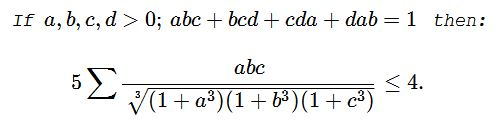# An Inequality with Constraint in Four Variables V

### Statement### Solution 1

By Hölder's inequality,

\displaystyle \begin{align} &(1+a^3)(1+b^3)(1+c^3)\ge (1+abc)^3\\ &\sqrt{(1+a^3)(1+b^3)(1+c^3)}\geq 1+abc\\ &\frac{1}{\sqrt{(1+a^3)(1+b^3)(1+c^3)}}\leq \frac{1}{1+abc} \end{align}

It follows that

\displaystyle \begin{align} \sum_{cycl}\frac{abc}{\sqrt{(1+a^3)(1+b^3)(1+c^3)}}&\leq \sum_{cycl}\frac{abc}{1+abc}\\ &=\sum_{cycl}\frac{1+abc-1}{1+abc}\\ &=4-\sum_{cycl}\frac{1}{1+abc} \end{align}

$\displaystyle \sum_{cycl}\frac{1}{1+abc} \ge \frac(1+1+1+1)^2}4+\sum_{cycl}abc}=\frac{16}{5}$

Thus, $\displaystyle -\sum_{cycl}\frac{1}{1+abc}\leq -\frac{16}{5}.\,$ Further,

\displaystyle \begin{align} &4-\sum_{cycl}\frac{1}{1+abc}\leq 4-\frac{16}{5}=\frac{4}{5}\\ &\sum_{cycl}\frac{abc}{\sqrt{(1+a^3)(1+b^3)(1+c^3)}}\leq \frac{4}{5}\\ &5\sum_{cycl}\frac{abc}{\sqrt{(1+a^3)(1+b^3)(1+c^3)}}\leq 4. \end{align}

### Solution 2

By Hölder's inequality, $\displaystyle \sqrt{\prod_{cycl}(1+a^3)}\ge 1+abc,\,$ implying

$\displaystyle 5\sum_{cycl}\fracabc}\sqrt{\prod_{cycl}(1+a^3)}}\le5\sum_{cycl}\frac{abc}{1+abc}$

By Jensen's inequality applied to the concave function $\displaystyle f(x)=\frac{x}{1+x},\,$

$\displaystyle 5\sum_{cycl}\frac{abc}{1+abc}\le 20\frac{frac{1}{4}}{\frac{5}{4}}=4.$

### Acknowledgment

Dan Sitaru has kindly posted a problem of his from the Romanian Mathematical Magazine at the CutTheKnotMath facebook page and later emailed his solution in a LaTeX file. Solution 2 is by Leo Giugiuc. Arben Ajredini has independently came up with Solution 1.